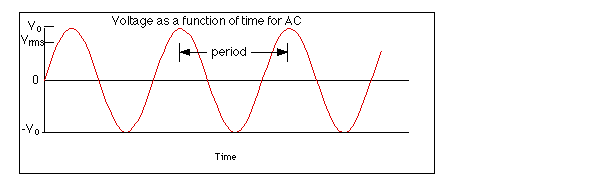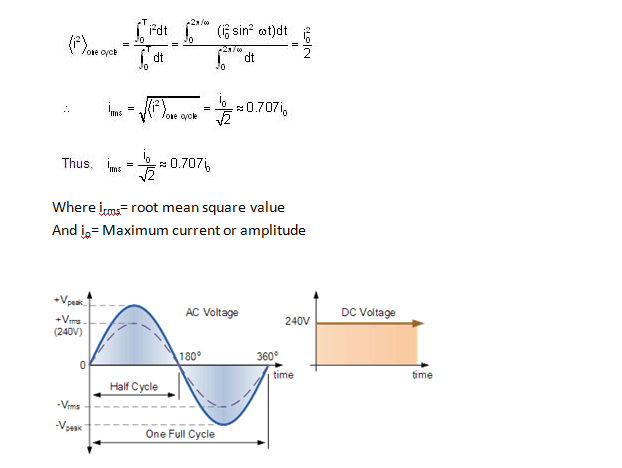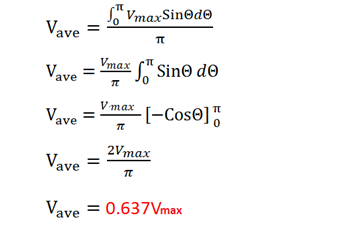Generally, A.c. generators produce emf which is sinusoidal. Thus, the relationship between the induced emf and the time taken is given by the following equation

e= EmaxSinωt

Where e= instantaneous induced voltage

Emax= maximum induced voltage

ωt= θ=angle at which the coil rotates with respect to the magnetic field

ω= 2πf, where ‘f’ is the frequency in hertz

e= Emax Sin2πft

1. Amplitude: The peak value of the alternating wave, whether positive or negative is referred to as its amplitude.As per the graph given above ‘Vo’ is the positive amplitude and ‘-Vo’ is the negative amplitude.
2. Cycle: In an alternating Wave one set of crest and trough is called as one cycle. Generally, one cycle amounts to the time period of the wave.
3. Time period: Time period generally refers to the total time taken by the alternating wave to complete one cycle.
4. Frequency: The number of cycles of alternating waves per second is called frequency. The SI unit of frequency is Hertz (Hz). Frequency is the reciprocal of the time period

f(Hertz)= 1/time period(second)

1. Root mean square(RMS) value: Root mean square value of an alternating current is defined as the value of the D.C. current which would have passed through the same circuit and has generated the same heat as it would have been generated by the A.C. current, for the same time period. In other words, it is also the effective value of the alternating current. Generally, millimeters, voltmeters, ammeter,etc. give the reading of RMS value for an alternating current.

The RMS value is the square root of the mean value of the obtained squared function for a cycle or half cycle. This value of RMS is generally used for all power calculation. The root mean square value of AC over one cycle is given by the following equation,1. Mean or Average value: Mean or Average value of an alternating current is defined as the value of the D.C. current which would have passed through the same circuit and has transferred the same amount of charge as it would have been transferred by the A.C. current, over the half cycle.The following equation represents the derived relation between the average value and the peak value,Average or the mean value of the alternating current or emf is ‘0’ over a cycle as the positive and negative values get canceled.

Links of Next Electrical Engineering Topics:-### Customer Reviews

My Homework Help
Rated 5.0 out of 5 based on 510 customer reviews at